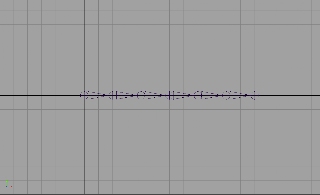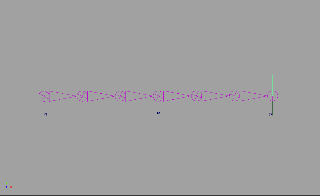## Wednesday, June 19, 2013

### Creating Stretchy IK Joint Chain

I thought it would be nice to go over how to create a basic yet so important setup in character rigging. The concept itself is quite simple, yet I've seen many times people struggle with the idea. So in this post I'll go over how to setup a stretchy joint chain being driven by an Ik solver using code. The code itself will be generating utility nodes and hooking things up for us to make the setup work.

If you want you can download a tool that uses this technique here

Watch instructional video here

From this hopefully you'll be able to use the information to create your own version of this setup. So lets get to it. We will be using the python maya.cmds package

`import maya.cmds as cmds`
For us to setup a stretchy joint chain, first we will require some joints.Joint Chain
Once the joints have been created all we really need is to specify what is the start and end of our stretchy joint chain. With a start and end joint we can go ahead an create a new Ik handle. For this demonstration we will use a single chain solver (SC). So lets define a method that takes in a start and an end joint. Lets also go ahead and also rename and store the new nodes created with the Ik handle so we can keep track of them.

```def stretchyIKChain(startJoint, endJoint):
ikNodes = cmds.ikHandle(sj=startJoint, ee=endJoint, solver="ikSCsolver", n=startJoint + "_ikHandle")
ikNodes = cmds.rename(ikNodes, startJoint + "_effector")
ikHandle = ikNodes
```

If we were to describe the process of making this joint chain stretch uniformly, we would say that we want each individual 'bone' to stretch by the same factor. That stretch factor would be the current length divided by the original length. So right from the start we already know we need a way to measure the length  from our start joint to the end joint. Thankfully we have something called the 'distance tool'. In maya, it is located under Create -> Measure Tools -> Distance Tool. We want to create a distance node measuring form the start joint to the end joint. The tool will create a locator at each end. So to do this in our code we will create locators and hook it up ourselves.

```    # create locators for distance tool node
startLocator = cmds.spaceLocator(n=startJoint + "_startLocator")
cmds.pointConstraint(startJoint, startLocator, mo=False, n=startLocator + "_pointConstraint")

endLocator = cmds.spaceLocator(n=endJoint + "_endLocator")
cmds.xform(endLocator, ws=True, absolute=True, translation=cmds.xform(ikHandle, q=True, ws=True, translation=True))
cmds.pointConstraint(endLocator, ikHandle, mo=False, n=ikHandle + "_pointConstraint")

distanceNode = cmds.createNode("distanceDimShape", n="%s_%s_distance" % (startLocator,endLocator))

cmds.connectAttr(startLocator + "Shape.worldPosition", distanceNode + ".startPoint")
cmds.connectAttr(endLocator + "Shape.worldPosition", distanceNode + ".endPoint")
```Ik Joint Chain distanc
The point constraint will serve 2 purposes. With 'maintainoffset' set to False it will snap the locator to the position we want, and now the start locator will follow the start joint. For the end locator we first move it to the end joints positions, where the ikHandle is, and point constraint the ikHandle to the locator. This way, you can organize the nodes and put the ikHandle in other group and just work with the locator. The locator will be your control for the stretchy ikHandle, you can parent it or constraint it to another control curve if would like to. Next, we hook up the locator to the distance node through their worldPosition attribute.

I mentioned we will be dividing the current length by the original length. The distance node provides with current distance between the locators, and because we have hooked up the locators to our ik joints, it will update correctly. However, we need to get the original length of the joint chain. We could just get the distance value right now, but what if the joint chain was laid out straight like we did it here. What if it was something like thisNon-straight Joint chain
Then the default distance doesn't actually represent the length of the joint chain. If we move the ikHandle so that the joints are lined up straight, we will then have the actual original length. Its from this point on that we want the joints to start stretching. But we won't do it this way.

One solution could be to require whoever is setting this up, to have the joints straighten out beforehand. But that's really inconvenient. The other, and much better alternative, is to find the total length of the joint chain by adding the translateX values of all child joints starting with the start joint's first child all the way to the end joint. Now I said translateX because by default the x-axis is the joint's rotation axis, the one going down the joint. But what if that's not the case. Then need first a way to find the rotation axis. To do that we just need to check any of the child joint (we will use the end joint) for its translations and whichever axis has a value, that axis is the rotation axis. This is how that would look like

```def getRotationAxis(joint):
''' Get the rotation axis from the translations in the child joint '''
# get the translation values of the child joint
translate = cmds.getAttr(joint + ".t")
# to store the axis string
axis = ""
for i, t in enumerate(translate):
# check which translate axis has non-zero values
# check for + and - values. Joints could be going opposite direction
value = abs(t)
if value > .0001:
if i == 0:
axis = "x"
elif i == 1:
axis = "y"
elif i == 2:
axis = "z"
if not axis:
cmds.error("Child joint is too close to its parent.")
return axis
```

Now, that we have a way to determine the ration axis lets use it to find the original length of our joint chain.

```    rotationAxis = getRotationAxis(endJoint)

# to store original joint chain length
originalLength = 0.0

# to store child joints
childJoints = []

currentJoint = startJoint
done = False

while not done:
# get the child joints for the current joint
children = cmds.listRelatives(currentJoint, c=True)
children = cmds.ls(children, type="joint")

# we reached the end of the joint chain
if not children:
done = True
else:
child = children
childJoints.append(child)

# start summing the original length. Add the absolute for + and - translate values
originalLength += fabs(cmds.getAttr(child + ".t" + rotationAxis))

currentJoint = child

# we reached the end of the stretchy joint chain
if child == endJoint:
done = True
```

Now that we have the original length we can find the stretchFactor by dividing the current distance by the  original length. We can use a 'multiplyDivide' node for this

```     # divide distance by original length
stretchFactorNode = cmds.createNode("multiplyDivide", n=ikHandle + "_stretchFactor")

cmds.setAttr(stretchFactorNode + ".operation", 2) # Divide
cmds.connectAttr(distanceNode + ".distance", stretchFactorNode + ".input1X")
cmds.setAttr(stretchFactorNode + ".input2X", originalLength)
```

Now that we have a normalized distance we can use it to stretch the joints. We can stretch the joints in two different ways. We can stretch them by driving the scale or by driving the translate of the child joints. To stretch using scale we can connect the output of the multiplyDivide node (stretchFactor) to the scaleX (or whatever our rotation axis is) of all joints except the end joint.

```    # connect joints to stretchy math nodes using scale
childJoints.insert(0, startJoint)
childJoints.remove(endJoint)

for joint in childJoints:

multiplyNode = cmds.createNode("multiplyDivide", n=joint + "_translate_stretchMultiply")

cmds.setAttr(multiplyNode + ".input1X", cmds.getAttr(joint + ".t" + rotationAxis))
cmds.connectAttr(stretchFactorNode + ".outputX", multiplyNode + ".input2X")
cmds.connectAttr(multiplyNode + ".outputX", joint + ".t" + rotationAxis)
```

Nice! We are finally done setting up our stretchy joints using scale. Now lets look at how to do it for translate. The idea is the same, but with translate we want to multiply the joint's original translate by our stretchFactor instead of just plugging it in. We will do this for all joints except the first the start joint. So lets do that.

```    # connect joints to stretchy math nodes using translate
for joint in childJoints:

multiplyNode = cmds.createNode("multiplyDivide", n=joint + "_translate_stretchMultiply")

cmds.setAttr(multiplyNode + ".input1X", cmds.getAttr(joint + ".t" + rotationAxis))
cmds.connectAttr(stretchFactorNode + ".outputX", multiplyNode + ".input2X")
cmds.connectAttr(multiplyNode + ".outputX", joint + ".t" + rotationAxis)
```

Awesome! We now have a stretchy joint chain! So now you could even add the option to the stretchyIK function to allow the user to pick between scale and translate setup. I'll set the default method to translate.

```def stretchyIKChain(startJoint, endJoint, stretchMethod="translate")
```

So, are we done? Well, our joints certainly stretch now when we pull on the ikHandle. But what if we move the ikHandle towards the start joint. Oh-oh! That's not good. The preferred angle on the joints are neglected and we are now overriding the joints, and they are now actually squashing instead of maintaining their original length. If you want that effect, well you don't need an ikHandle in the first place. And now you know how to set it up to have stretchy joints that squash and stretch. With what you've learned here. Its really easy to create an expression to do that for us.

In fact, I might go over in another post, how to create stretchy joints using expressions. But, going back to where we were, we want to have the joints only stretch when the distance is greater than or equal to the original length. So how do we do that using utility nodes? Using a 'condition' node! Like this

```    # create a condition node to allow stretching only when the distance is >= the original length
conditionNode = cmds.createNode("condition", n=startJoint + "_condition")

cmds.setAttr(conditionNode + ".operation", 3) # >=

cmds.setAttr(conditionNode + ".firstTerm", originalLength)
cmds.setAttr(conditionNode + ".colorIfTrueR", originalLength)
cmds.connectAttr(distanceNode + ".distance", conditionNode + ".secondTerm", f=True)
cmds.connectAttr(distanceNode + ".distance", conditionNode + ".colorIfFalseR", f=True)
```

Now we just need to make sure this code comes before the one where we create and hook up the 'stretchFactorNode' (multiplyDivide). And we will need to change that code for this

```# divide distance by original length
stretchFactorNode = cmds.createNode("multiplyDivide", n=ikHandle + "_stretchFactor")

cmds.setAttr(stretchFactorNode + ".operation", 2) # Divide
cmds.connectAttr(conditionNode + ".outColorR", stretchFactorNode + ".input1X")
cmds.setAttr(stretchFactorNode + ".input2X", originalLength)
```

Great! Now we can say we are officially done! Go and create your own stretchy IK joints!